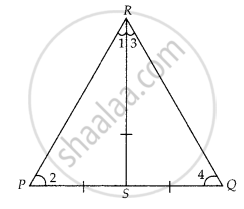# If S is a point on side PQ of a ΔQR such that PS = QS = RS, then ______. - Mathematics

MCQ
Fill in the Blanks

If S is a point on side PQ of a ΔQR such that PS = QS = RS, then ______.

#### Options

• PR . QR = RS2

• QS2 + RS2 = QR2

• PR2 + QR2 = PQ2

• PS2 + RS2 = PR2

#### Solution

If S is a point on side PQ of a ΔQR such that PS = QS = RS, then PR2 + QR2 = PQ2.

Explanation:

Given, in ∆PQR,

PS = QS = RS  .......(i)

In ∆PSR, PS = RS  ......[From equation (i)]

⇒ ∠1 = ∠2  .......(ii)

[Angles opposite to equal sides are equal]Similarly, in ∆RSQ, RS = SQ

⇒ ∠3 = ∠4  .......(iii)

[angles opposite to equal sides are equal]

Now, in ∆PQR, sum of angles = 180°

⇒ ∠P + ∠Q + ∠P = 180°

⇒ ∠2 + ∠4 + ∠1 + ∠3 = 180°

⇒ ∠1 + ∠3 + ∠1 + ∠3 = 180°

⇒ 2(∠1 + ∠3) = 180°

⇒ ∠l + ∠3 = 180∘2 = 90°

∴ ∠R = 90°

In ∆PQR, by Pythagoras theorem,

PR2 + QR2 = PQ2

Concept: Application of Pythagoras Theorem in Acute Angle and Obtuse Angle
Is there an error in this question or solution?

#### APPEARS IN

NCERT Mathematics Exemplar Class 10
Chapter 6 Triangles
Exercise 6.1 | Q 12 | Page 62

Share